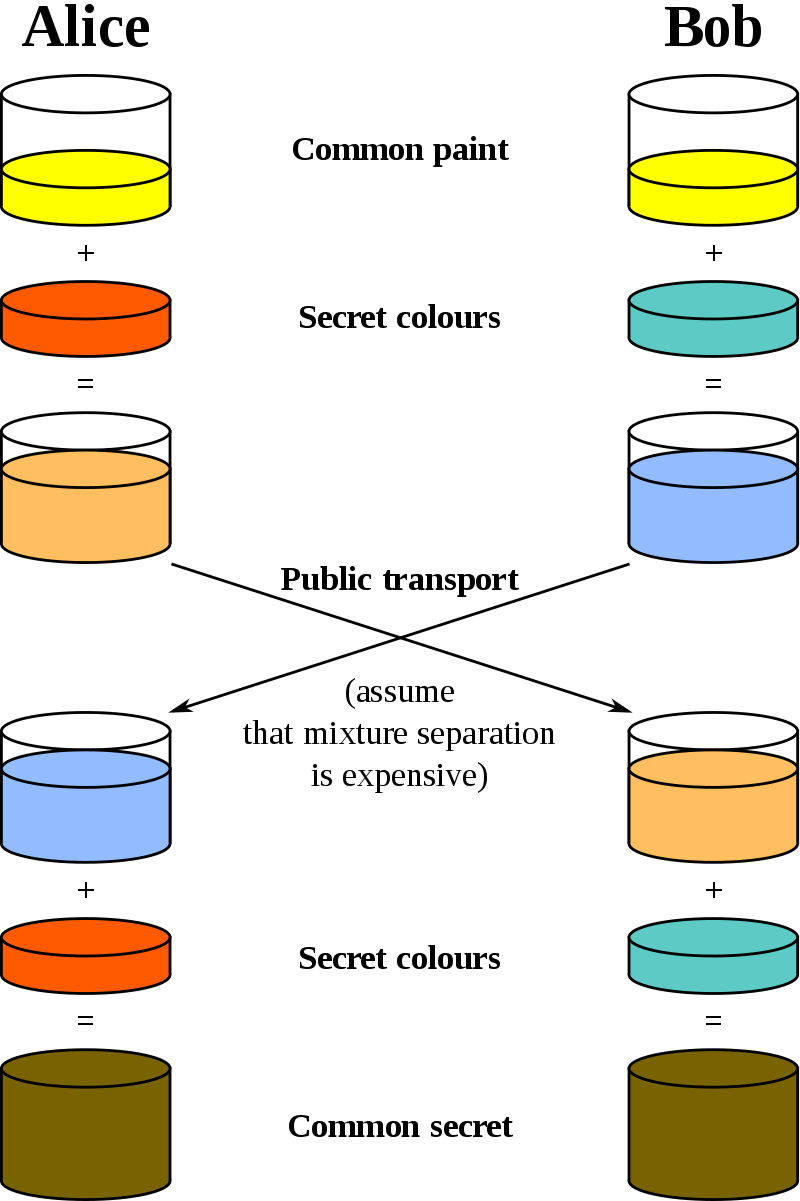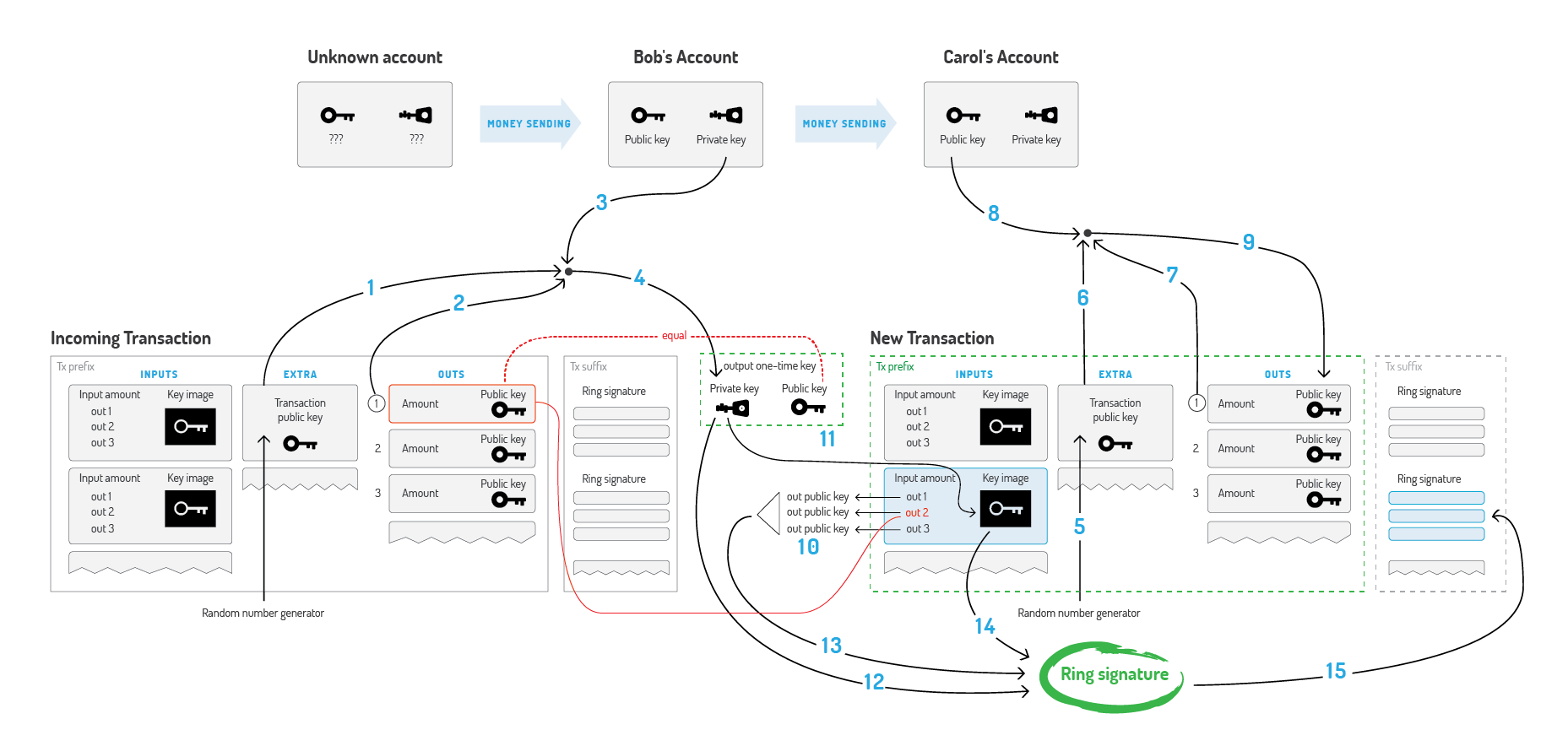$$r\xrightarrow{\times G} R, but\quad R\stackrel{\div G}\nrightarrow r$$

Alice：我們先選出一個大數G，這個G所有人都可以知道。然後我在心裡面想一個大數a，我把a乘以G的結果，假設是A告訴Bob，當然A是多少也被大家聽到了。

Bob：好的，現在我也在心裡想一個大數b，並且把b乘以G的結果B告訴Alice。

Alice：明白了，那麼最終敲定的金鑰就是a乘以B的結果，這個用計算機很快就能算出來。

Bob：算出來的結果不用告訴我，因為我拿b乘以A得到的結果是一樣的。

$$(g^a\;mod\;p)^{b}\;mod\;p=(g^b\;mod\;p)^{a}\;mod\;p$$，其中p是素數，g是p的本原根。1. Alice將m編碼後計算：M=m+aB。
2. Bob拿到M計算：m=M-bA，完成。

1. Bob在鏈上公開了他的標準地址，這個地址不是直接用於收款的，而是儲存了兩個公鑰（$$B_1、B_2$$），任何人都能獲得，他自己則保留對應的兩個私鑰（$$b_1、b_2$$）。$$B_1=b_1G,B_2=b_2G$$,這裡G是橢圓曲線上選取的基點，全網統一，同樣的演算法基點都是一樣的.
門羅標準地址格式：網路編碼(1 byte)+public spend key(32 byte)+public view key(32 byte)+校驗和(4 byte)
2. Alice隨機生成了一個整數r作為另一個私鑰，套用公式$$P=H_s(rB_1)G+B_2$$得[一次性]公鑰P，其中$$H_s$$是一個雜湊函式。P就是Bob的一次性收款地址（stealth address）。
思考$$H_s$$的作用？
3. Alice使用P作為轉賬的目標地址，同時將$$R=rG$$打包進此次交易。注意她可以使用相同的r進行不同目標地址的轉賬。
4. Alice將交易傳送至鏈，因此P、R也一同被公開到鏈上。
P中的$$H_s$$不但規範了P的資料結構（長度），同時也避免第三方將P與Bob關聯起來。試想，若無$$H_s$$，那麼$$P=rB_1G+B_2=RB_1+B_2$$，第三方很容易通過試算得到實際的收款方。
5. Bob掃描區塊鏈上[自上次掃描以來的]最近交易，計算$$P'=H_s(b_1R)G+B_2$$。如果P’=P，則說明這筆轉賬是給Bob的，因為$$b_1R=b_1rG=rb_1G=rB_1$$。
6. Bob要花這筆錢（轉賬給別人），則首先要計算出與P對應的私鑰x，即xG=P，由$$P=P'=H_s(b_1R)G+B_2$$可得：$$x=H_s(b_1R)+b_2$$。這個私鑰連給錢的Alice也無法推出（因為有$$b_2$$項），日後Bob就可以使用這個私鑰來花這筆錢了。

1. 隨機選取n-1個公鑰，連同自己的公鑰$$P_s$$組成n個公鑰集合$$\{P_1,P_2,\cdots ,P_s\cdots ,P_n\}$$；
2. 隨機選取n-1個值$$\{x_1,x_2,\cdots ,x_n\}$$，其中$$x_s$$暫缺，後面會使用計算得到的合適值補上；
這兩步和之前的例子一樣。
3. 設一未知數$$v_1$$，再設$$Y_1=x_1G+v_1P_1$$；
4. 設一未知數$$l$$，再設$$R_1=x_1H_p(P_1)+v_1l$$；
注意$$l,R_i$$存在與否並不影響環簽名，而是另有考慮，可看下節Key Image，此節可忽略。
5. 計算$$v_2=H_s(m,Y_1,R_1)$$，其中$$H_p$$和$$H_s$$是兩個不同的雜湊函式，m是需要簽名的資料，根據業務需求來；
6. 同上依次計算$$Y_2=x_2G+v_2P_2;R_2=x_2H_p(P_2)+v_2l;v_3=H_s(m,Y_2,R_2);\cdots ;Y_n=x_nG+v_nP_n;R_n=x_nH_p(P_n)+v_nl;v_{n+1}=H_s(m,Y_n,R_n)$$；
7. 令$$\underline{v_{n+1}=v_1}$$，這就是我們要構造的方程，為了使方程成立，關鍵落在尋找合適的$$x_s$$及未知數$$v_1,l$$上；
8. 雖然每一環的$$v$$由上一環而來，但是由於其首尾相連，我們無法由任一環推出其值，也就是說其值是不定/任意的；那麼我們可以先隨意設定某環的計算結果，再反推出符合該結果的$$v$$值。因此任選一個數a，使得$$Y_s=aG,R_s=aH_p(P_s)$$，$$Y_s,R_s$$一旦確定，$$v_{s+1}=H_s(m,Y_s,R_s)$$也跟著確定下來，繼而$$v_{s+2},v_{s+3},\cdots ,v_n,v_1,\cdots ,v_{s}$$都可依次推得；
9. 又$$Y_s=x_sG+v_sP_s$$，易得$$x_s=a-v_ss_s$$，其中$$s_s$$是$$P_s$$對應的私鑰。如此，$$\mathbb{X}$$被補足，現在只剩$$l$$未知；
10. 由$$R_s=x_sH_p(P_s)+v_sl=aH_p(P_s)$$及$$a=x_s+v_ss_s$$，易得$$l=s_sH_p(P_s)$$。
11. 證明方將$$\mathbb{P}$$、$$\mathbb{X}$$、$$v_1$$、$$l$$公開到鏈上，作為交易的發起者；礦工驗證。

Key Image

monero也使用UTXO，畢竟匿名性就決定了交易/餘額不能基於賬戶模型，但與比特幣不同，由於環簽名的緣故，礦工無法確定一個交易中的實際UTXO是哪個，自然無法將其從資料庫中標記刪除，從這個角度說，UTXO一旦產生，就永遠有效，這樣顯然不合理。門羅幣使用Key Image解決這個問題。簡單來說，一個Key Image對應一個UTXO（UTXO被花費的同時產生對應的Key Image），礦工只要檢視這次交易產生的Key Image是否存在，即可判斷UTXO是否被重複消費。上述的$$l$$就承擔了Key Image的職責。

Alice 在給 Bob傳送XMR的時候，其實是將自己的一筆或多筆UTXO變成Bob的一筆UTXO（當然一般還包括礦工費及找零，此處忽略）；

2017年1月10日Monero中正式使用了RingCTs。而在此之前，Monero中假如Alice需要向Bob轉12.5個XMR，則需要將自己的UTXO分別傳送至三個地址，分別轉賬10XMR、2XMR和0.5XMR，這是因為Monero中要求轉賬的XMR格式為A×10BA×10B的格式，環簽名中誘騙的UTXO也要相同數額。使用了RingCTs之後，在區塊鏈上隱藏了交易數額，這時候Alice挑選mixins的時候，不用考慮mixins的XMR的餘額，提升了交易的隱私性。

Alice需要花費的真實UTXO有幾筆，該過程就重複幾次。Ring Confidential Transactions

OK，目前為止，我們實現了在一次轉賬中：

• 隱藏轉賬方[UTXO[一次性]公鑰地址]（環簽名）。
• 隱藏了收款方[標準公鑰地址]（一次性地址）。
• 防止雙花（Key Image）。

1. 首先，輸入=輸出。這很好理解，Alice支出的錢必須得等於(Bob收到的錢+礦工費+找零)，收支平衡，只要保證貨幣初始來源合法（如挖礦），那麼追根溯源，後續的UTXO必然合法，然而這還不夠。
2. 假如Alice構造了一筆交易輸入=1BTC，輸出=1000BTC+(-999)BTC，不考慮礦工費，其中1000BTC轉給自己的另一個地址，-999BTC轉給原地址作為找零，後續她可以不管原地址，而使用新地址，如此則憑空多出了鉅額財富。要避免這種情況很簡單，只要規定(每筆輸入輸出>0)。

Pedersen commitments

C_{in}=xG+aG\Longrightarrow \left\{\begin{aligned}C_{out-1}=y_1G+b_1G\\C_{out-2}=y_2G+b_2G\end{aligned}\right.。

monero構造上述承諾稍有不同，留待以後講解。

1. 將金額b表示成二進位制形式：$$b=b_02^0+b_12^1+\cdots +b_n2^n,\ b_i\in \{0,1\}$$；
2. 將私鑰y對應拆分為n個隨機數之和：$$y=y_0+y_1+\cdots +y_n$$；
3. 根據加法同態，C可表示為：$$C=C_0+C_1+\cdots +C_n$$，其中$$C_i=y_iG+(b_i2^i)H$$；
4. 針對每個私鑰$$y_i$$，取兩個公鑰$$\{C_i,C_i-2^iH\}$$，兩者必有一個等於$$y_iG$$，所以真正的金鑰對為$$<y_i,y_iG>$$。將該公鑰對進行環簽名形成二元環簽名，表明構造者持有這兩個公鑰的其中一個私鑰。有多少個私鑰，就對應多少個二元環簽名；
5. 礦工只需進行兩步驗證：1、$$C_i$$之和是否等於C；2、各個環簽名是否正確。

https://cryptonote.org/whitepaper.pdf

Ring signature  環簽名（Ring signature）